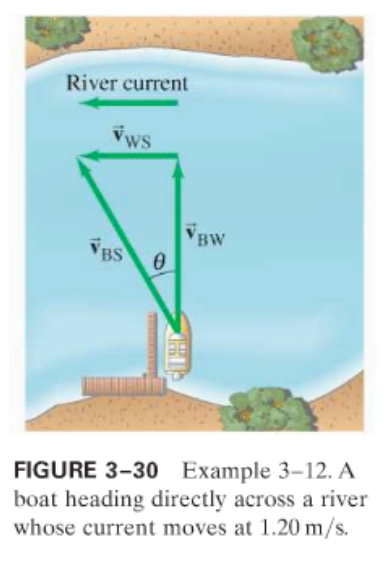×
Get Full Access to Physics: Principles With Applications - 6 Edition - Chapter 3 - Problem 39p
Get Full Access to Physics: Principles With Applications - 6 Edition - Chapter 3 - Problem 39p

×

# A boat can travel 2.30 m/s in still water. (a) If the boatISBN: 9780130606204 3

## Solution for problem 39P Chapter 3

Physics: Principles with Applications | 6th Edition

• Textbook Solutions
• 2901 Step-by-step solutions solved by professors and subject experts
• Get 24/7 help from StudySoup virtual teaching assistantsPhysics: Principles with Applications | 6th Edition

4 5 1 332 Reviews
19
5
Problem 39P

A boat can travel $$2.30 \mathrm{~m} / \mathrm{s}$$ in still water.

(a) If the boat points its prow directly across a stream whose current is $$1.20 \mathrm{~m} / \mathrm{s}$$, what is the velocity (magnitude and direction) of the boat relative to the shore?

(b) What will be the position of the boat, relative to its point of origin, after 3.00 s? (See Fig. 3–30.)Equation Transcription:Text Transcription:

2.30 m/s

1.20 m/s

Step-by-Step Solution:

Step 1 :In this question , a particle is rotating in circle with centripetal acceleration ofa = 8.0 m/s 2We need to infer what would happen if we double the radius of the circle withoutchanging its speedIn the second scenario we need find what would happen if we double the speed of theparticle without changing the radius of the circle

Step 2 of 4

Step 3 of 4

##### ISBN: 9780130606204

This textbook survival guide was created for the textbook: Physics: Principles with Applications, edition: 6. Since the solution to 39P from 3 chapter was answered, more than 519 students have viewed the full step-by-step answer. Physics: Principles with Applications was written by and is associated to the ISBN: 9780130606204. The answer to “?A boat can travel $$2.30 \mathrm{~m} / \mathrm{s}$$ in still water. (a) If the boat points its prow directly across a stream whose current is $$1.20 \mathrm{~m} / \mathrm{s}$$, what is the velocity (magnitude and direction) of the boat relative to the shore? (b) What will be the position of the boat, relative to its point of origin, after 3.00 s? (See Fig. 3–30.) Equation Transcription: Text Transcription:2.30 m/s 1.20 m/s” is broken down into a number of easy to follow steps, and 71 words. This full solution covers the following key subjects: boat, relative, current, across, directly. This expansive textbook survival guide covers 35 chapters, and 3914 solutions. The full step-by-step solution to problem: 39P from chapter: 3 was answered by , our top Physics solution expert on 03/03/17, 03:53PM.

## Discover and learn what students are asking

Calculus: Early Transcendental Functions : Differential Equations: Growth and Decay
?In Exercises 1-10, solve the differential equation. $$\frac{d y}{d x}=6-y$$

Calculus: Early Transcendental Functions : Second-Order Homogeneous Linear Equations
?Finding a General Solution In Exercises 5-30,find the general solution of the linear differential equation. y’' - y’ - 6y = 0

Statistics: Informed Decisions Using Data : Tests for Independence and the Homogeneity of Proportions
?Dropping a Course A survey was conducted at a community college of 50 randomly selected students who dropped a course in the current semester to learn

Unlock Textbook Solution